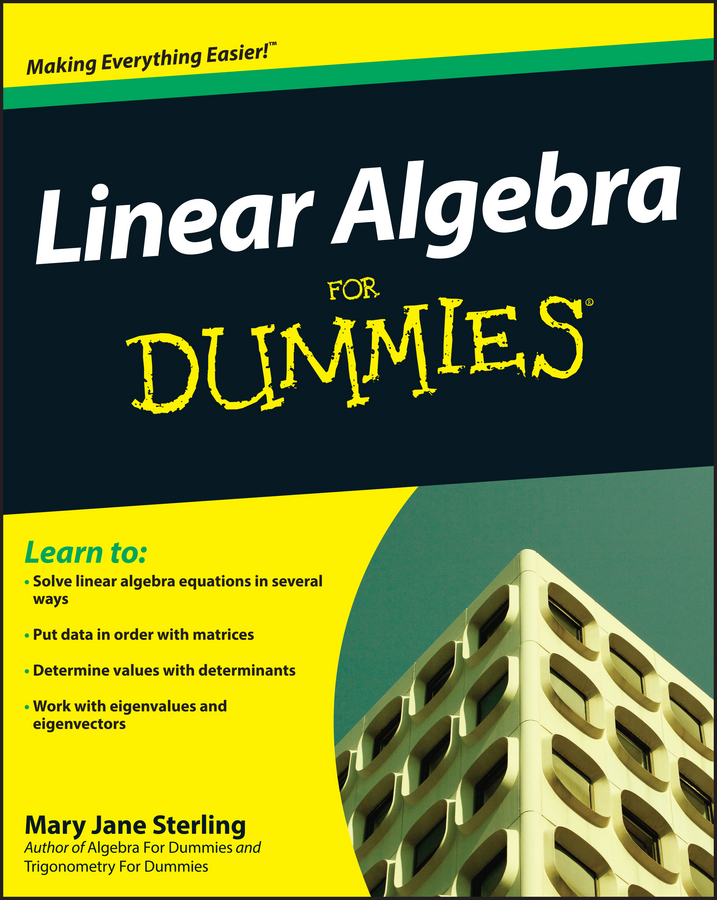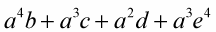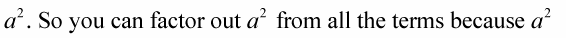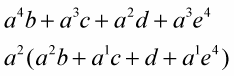##### Linear Algebra For DummiesYou can factor out variables from the terms in an expression. You factor out variables the same way as you do numbers except that when you factor out powers of a variable, the smallest power that appears in any one term is the most that can be factored out.

Variables represent values; variables with exponents represent the powers of those same values.

In an expression such asthe smallest power of a that appears in any term isis the greatest common factor. You can’t factor anything else out of each term:Check your work quickly by doing either of the following: